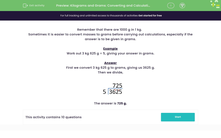# Kilograms and Grams: Converting and Calculating

In this worksheet, students carry out calculations on weights giving their answers in grams.Key stage:  KS 2

Curriculum topic:   Measurement

Curriculum subtopic:   Solve Four Operations Problems

Difficulty level:#### Worksheet Overview

Remember that there are 1000 g in 1 kg.

Sometimes it is easier to convert masses to grams before carrying out calculations, especially if the answer is to be given in grams.

Example

Work out 3 kg 625 g ÷ 5, giving your answer in grams.

First we convert 3 kg 625 g to grams, giving us 3625 g.

Then we divide,